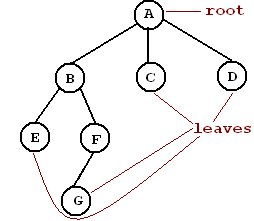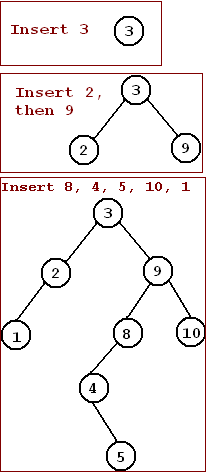# Lab 8: Binary Search Trees I

## Objectives

Learn how to implement the tree data structure.

## Trees

• Definition: A tree is a collection of nodes. The collection consists of one node is distinguished as the root and zero or more subtrees whose roots are connected by a directed edge to the root (p. 113)
• Terminology pp. 113-114• Root: a distinguished node from which all subtrees proceed.
• Edge: A line joining two nodes in a tree. The line is directed; that is, it can be traveled in only one direction (down), e.g., there is an edge from A to B, but not from B to A.
• Child: A node n2 is a child of node n1 if there is an edge from n1 to n2, e.g., F is a child of B. B is the Parent of F.
• Siblings: Two nodes are siblings if they share the same parent, e.g., B, C, and D are siblings, as are E and F.
• Leaf: a leaf is a node with no children.
• Path: a path from node n1 to nk is defined as a sequence of nodes n1, n2, . . ., nk such that ni is the parent of ni+1 for 1 <= i < k, e.g., there is a path of length 3 from A to G: ABFG. There is NO path from C to B.
• Ancestor a node n1 is an ancestor to node n2 if there is a path from n1 to n2. If this is the case, then n2 is a descendent of n1.
• Depth: a node n1's depth is the length of the path (number of edges) from the root to n1, e.g., the depth of E is 2.
• Height: The height of a tree is the longest path from the root to a leaf, e.g., the height of this tree is 3.

## Binary Search Trees pp. 124-130

• Definition: A binary tree (a tree in which no node can have more than two children) with the following property: for every node n in the tree, the values of all nodes in n's left subtree are smaller than n, and the values of the nodes in n's right subtree are greater than n.
• Uses: Quick searches of nodes (O log2N) and sorting of data.
• Building a tree: consider adding the following values to a tree: 3 9 2 8 4 5 10 11. Insert 3: Since the tree is empty, 3 becomes the root.
2. Insert 9: Compare 9 to 3. 9 > 3. Insert 9 to the right of 3.
3. Insert 2: Compare 2 to 3. 2 < 3. Insert 2 to the left of 3.
4. Insert 8: Compare 8 to 3. 8 > 3. Insert 8 to the right of 3.
Compare 8 to 9. 8 < 9. Insert 8 to the left of 9.
5. Insert 4: Compare 4 to 3. 4 > 3. Insert 4 to the right of 3.
Compare 4 to 9. 4 < 9. Insert 4 to the left of 9.
Compare 4 to 8. 4 < 8. Insert 4 to the left of 8.
6. Insert 5: Compare 5 to 3. 5 > 3. Insert 5 to the right of 3.
Compare 5 to 9. 5 < 9. Insert 5 to the left of 9.
Compare 5 to 8. 5 < 8. Insert 5 to the left of 8.
Compare 5 to 4. 5 > 4. Insert 5 to the right of 4.
7. Insert 10: Compare 10 to 3. 10 > 3. Insert 10 to the right of 3.
Compare 10 to 9. 10 > 9. Insert 10 to the right of 9.
8. Insert 1: Compare 1 to 3. 1 < 3. Insert 1 to the left of 3.
Compare 1 to 2. 1 < 2. Insert 1 to the left of 2.

• Implementation
• The `tree_t` class stores a link to the root node.
• The root node has a data value, a link to a left child, and a link to a right child.
• Similarly, every other node in the tree has data, a left link, and a right link. If the node does not have a child, the link is `NULL`.

## Assignment

1. Provide the implementation (`tree.cpp`) for the following `tree_t` interface (`tree.h`):
``````#ifndef TREE_H
#define TREE_H

// forward declcarations
template <typename T> class tree_t;
template <typename T> std::ostream& operator<<(std::ostream&, const tree_t<T>&);

template <typename T>
class tree_t {

private:
struct node_t    // an all public class with data only, no member ftns
{
T        data;
node_t   *left;
node_t   *right;

node_t(const T& d = T(), node_t *l = NULL, node_t *r = NULL) : \
data(d), left(l), right(r) \
{ };
};

public:
tree_t();

// copy constructor
//tree_t(const tree_t& rhs);

// destructors
~tree_t()                           { clear(); }

// friends -- note the extra <> telling the compiler to instantiate
// a templated version of the operator<< -- <T> is also legal,
// i.e.,
// friend std::ostream& operator<< <T>(std::ostream& s, const tree_t&);
friend
std::ostream& operator<< <>(std::ostream& s, const tree_t& rhs);
friend
std::ostream& operator<<(std::ostream& s, tree_t *rhs)
{ return(s << (*rhs)); }
void inorder(std::ostream& s, node_t* const &t) const;

// assignment operator
const tree_t& operator=(const tree_t&);

// operators

// members
bool     empty() const              { return root == NULL ? true : false;}
bool     contains(const T& x) const { return contains(x,root); }
void     insert(const T& x)         { insert(x, root); }
void     clear()                    { clear(root); }

private:
node_t   *root;

bool     contains(const T&, node_t* ) const;
void     insert(const T& x, node_t* &t);
void     clear(node_t* &);
node_t*  clone(node_t* ) const;
};
#endif
``````
Details
• Recursive functions: private `clear`, `insert`, and `inorder` are recursive methods. That is, in order to make a node empty, you must first make its subtrees empty. Thus, `clear` is called recursively on the subtrees first before deleting the current node (if it is not `NULL` already). Similarly, `insert` is called recursively on subtrees to find the correct insertion point.
In order to start the recursion, the public methods `clear`, `insert`, and `operator<<` call the private versions with the root as the starting point.
• References to pointers: `insert` uses a reference to a pointer. The reason that we need to be able to change where the node points when we insert (e.g., if the node points to `NULL`, we need to change it to point to the new `node_t` we are inserting). We could use a pointer to a pointer; however, C++'s references allow simpler notation.
• The private `clear` method:
• This internal method removes the node passed in and its descendents.
• Before deleting the node, we call `clear` on its children.
• After the children have been made empty, we delete this node and set it to `NULL`. Setting the node to `NULL` is effective outside of the method call because the node passed in as a reference to a pointer.
• The private `insert` method (3 cases):
• If the node we're inserting to is `NULL`, insert this value as that node.
• Otherwise, if this value is < the value at the current node, call insert to the left child.
• Otherwise, insert to the right child.
• The private `inorder` method (inorder printing):
1. Call `inorder` on the left child.
2. Output the value of the current node.
3. Call `inorder` on the right child.
2. Test code with the following main function: ```#include <iostream> #include <cstdlib> #include <sys/time.h> #include "pairs.h" #include "tree.h" int main(); int main() { int num; tree_t<int> tree; tree_t<pairs_t> ptree; struct timeval tp; // get time of day gettimeofday(&tp,NULL); // use microseconds as the seed //srand((unsigned int)tp.tv_usec); srand((unsigned int)1337); // sort while inserting, thus maintaining list as priority queue std::cout << "Inserting:" << std::endl; for(int i=0;i<10;i++) { num = static_cast<int>((float)rand()/(float)RAND_MAX*100.0); std::cout << num << " "; tree.insert(num); } std::cout << std::endl; tree.insert(57); std::cout << "Tree contains 57? " << tree.contains(57) << std::endl; std::cout << "Tree: " << tree << std::endl; ptree.insert(pairs_t(45.0,'b')); ptree.insert(pairs_t(37.2,'c')); ptree.insert(pairs_t(42.6,'a')); ptree.insert(pairs_t(53.7,'d')); std::cout << "Pair Tree: " << ptree << std::endl; } ```

## Sample output

```Inserting: 1 86 24 21 30 74 89 64 21 68 Tree contains 57? 1 Tree: 1 21 24 30 57 64 68 74 86 89 Pair Tree: ('a',42.6) ('b',45) ('c',37.2) ('d',53.7) ```

## Turn in

Turn in all of your code, in one `tar.gz` archive of your lab##/ directory, including:
1. A `README` file containing
1. Course id--section no
2. Name
3. Lab description
4. Brief solution description (e.g., program design, description of algorithm, etc., however appropriate).
5. Lessons learned, identified interesting features of your program
6. Any special usage instructions
2. `Makefile`
3. source code (`.h` headers and `.cpp` source)
4. object code (do a `make clean` before `tar`)

## How to hand in

See `sendlab` notes